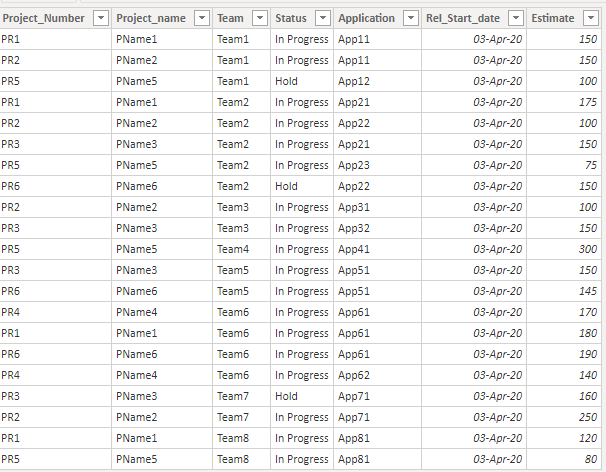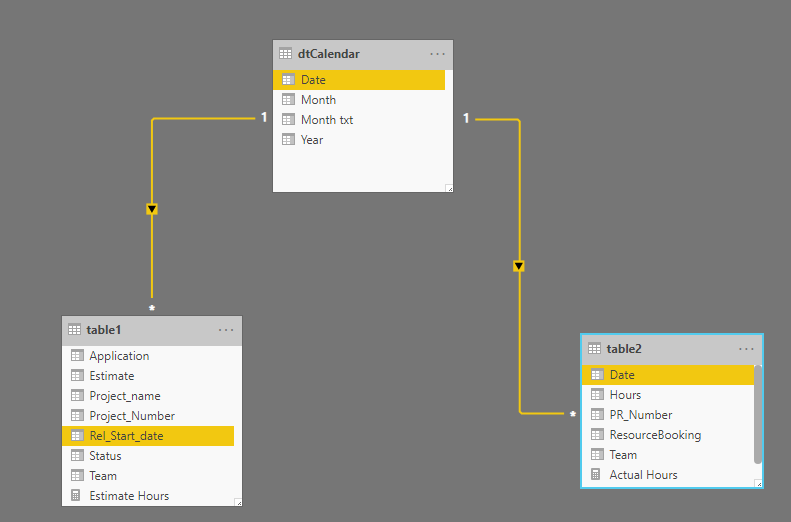Search

# Virtual Relationships with TREATASHandling relationships is an essential aspect of any data modeling exercise. If done correctly, it can save a lot of time and effort. Though Power BI offers many-to-many relationships, but at times it adds many complexities. And hence, experts advise caution while using many-to-many relationships in Power BI.

TREATAS offers a relatively simple solution in this case. Let us understand this by the following example.

Following are two sample tables:

Table 1:Table 2:## Objective

Calculate the Estimate vs. Actual report for each Project & Team.

As we notice, both the Project Number and Team have multiple values in both the table. If we establish a relationship, it is many-to-many.

For this exercise, we do not establish any relationship between the two tables

We have added a Calendar table and linked the two tables on DateRefer to the following article for adding a Calendar table in the data model

## Creating Basic Calculations

Added two measures for getting the total of Estimate and Actual Hours

``` Estimate Hours = SUM(table1[Estimate])

Actual Hours = SUM(table2[Hours])
```

## Creating Measures using TREATAS

TREATAS applies the result of a table expression as filters to columns from an unrelated table. It returns a table containing all the rows in column(s) that are in the expression.

``` Total Hours Team =
CALCULATE(
[Actual Hours],
TREATAS(VALUES(table1[Team]),table2[Team]),
TREATAS(VALUES(table1[Project_Number]),table2[PR_Number])
)
```

In the above example, TREATAS is doing the following:

· VALUES function creates a list of all unique Teams and Project Number from table1

· TREATAS creates a virtual many-to-one relationship on Teams and Project Number

## Output

I have used the measure in the table visual, which is producing the expected output